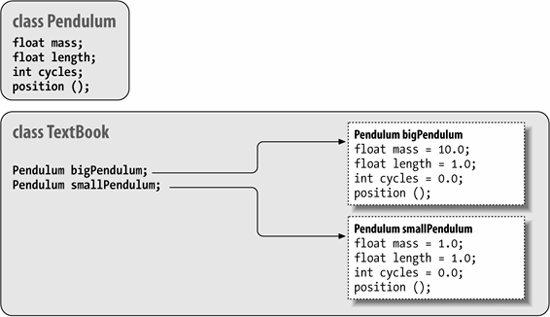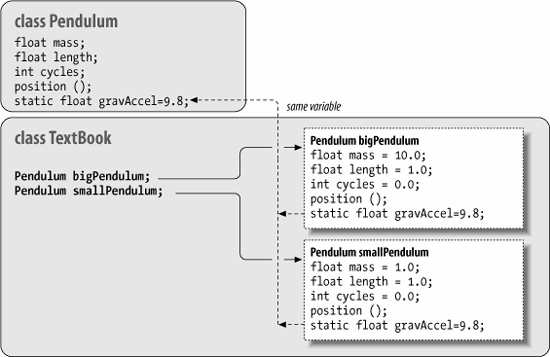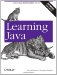# Section 5.1. Classes

### 5.1. Classes

Classes are the building blocks of a Java application. A class can contain methods (functions), variables, initialization code, and, as we'll discuss later, other classes. It serves as a blueprint for making class instances, which are runtime objects that implement the class structure. You declare a class with the class keyword. Methods and variables of the class appear inside the braces of the class declaration:

`     class Pendulum {         float mass;         float length = 1.0;         int cycles;         float getPosition ( float time ) {             ...         }         ...     }`

The Pendulum class contains three variables: mass, length, and cycles. It also defines a method called getPosition( ), which takes a float value as an argument and returns a float value as a result. Variables and method declarations can appear in any order, but variable initializers can't make "forward references" to other variables that appear later. Once we've defined the Pendulum class, we can create a Pendulum object (an instance of that class) as follows:

`     Pendulum p;     p = new Pendulum( );`

Recall that our declaration of the variable p doesn't create a Pendulum object; it simply creates a variable that refers to an object of type Pendulum. We still had to create the object, using the new keyword, as shown in the second line of the preceding code snippet. Now that we've created a Pendulum object, we can access its variables and methods, as we've already seen many times:

`     p.mass = 5.0;     float pos = p.getPosition( 1.0 );`

Two kinds of variables can be defined in a class: instance variables and static variables. Every object instance has its own set of instance variables; the values of these variables in one instance of an object can differ from the values in another object. We'll talk about static variables later, which, in contrast, are shared among all instances of an object. In either case, if you don't initialize a variable when you declare it, it's given a default value appropriate for its type (null, zero, or false).

Figure 5-1 shows a hypothetical TextBook application that uses two instances of Pendulum through the reference-type variables bigPendulum and smallPendulum. Each of these Pendulum objects has its own copy of mass, length, and cycles. As with variables, methods defined in a class may be instance methods or static methods. An instance method is associated with an instance of the class, but the relationship isn't quite as simple as for variables. Instance methods are accessed through an object instance, but the object doesn't have its own "copy" of the methods (there is no duplication of code). Instead, the association means that instance methods can "see" and operate on the values of the instance variables of the object. As you'll see in Chapter 6 when we talk about subclassing, there's more to learn about how methods see variables. In that chapter, we'll also discuss how instance methods can be "overridden" in child classesa very important feature of object-oriented design. Both aspects differ from static methods, which we'll see are really more like global functionsassociated with a class by name only.

#### 5.1.1. Accessing Fields and Methods

Inside a class, we can access variables and call methods of the class directly by name. Here's an example that expands on our Pendulum:

`     class Pendulum {         ...         void resetEverything( ) {             mass = 1.0;             length = 1.0;             cycles = 0;             ...             float startingPosition = getPosition( 0.0 );         }         ...     }`

##### Figure 5-1. Instances of the Pendulum classOther classes access members of an object through a reference, using the dot selector notation that we discussed in the last chapter:

`     class TextBook {         ...         void showPendulum( ) {             Pendulum bob = new Pendulum( );             ...             int i = bob.cycles;             bob.resetEverything( );             bob.mass = 1.01;             ...         }         ...     }`

Here we have created a second class, TextBook, that uses a Pendulum object. It creates an instance in showPendulum( ) and then invokes methods and accesses variables of the object through the reference bob.

Several factors affect whether class members can be accessed from another class. You can use the visibility modifiers public, private, and protected to control access; classes can also be placed into a package, which affects their scope. The private modifier, for example, designates a variable or method for use only by other members of the class itself. In the previous example, we could change the declaration of our variable cycles to private:

`     class Pendulum {         ...         private int cycles;         ...`

Now we can't access cycles from TextBook:

`     class TextBook {         ...         void showPendulum( ) {             ...             int i = bob.cycles;  // Compile-time error`

If we still need to access cycles in some capacity, we might add a public getCycles( ) method to the Pendulum class. (Creating accessor methods like this is a good design rule because it allows future flexibility in changing the type or behavior of the value.) We'll take a detailed look at packages, access modifiers, and how they affect the visibility of variables and methods in Chapter 6.

#### 5.1.2. Static Members

As we've said, instance variables and methods are associated with and accessed through an instance of the classi.e., through a particular object, like bob in the previous example. In contrast, members that are declared with the static modifier live in the class and are shared by all instances of the class. Variables declared with the static modifier are called static variables or class variables; similarly, these kinds of methods are called static methods or class methods. We can add a static variable to our Pendulum example:

`     class Pendulum {         ...         static float gravAccel = 9.80;         ...`

We have declared the new float variable gravAccel as static. That means if we change its value in any instance of a Pendulum, the value changes for all Pendulum objects, as shown in Figure 5-2.

Static members can be accessed like instance members. Inside our Pendulum class, we can refer to gravAccel like any other variable:

`     class Pendulum {         ...         float getWeight ( ) {             return mass * gravAccel;         }         ...     }`

However, since static members exist in the class itself, independent of any instance, we can also access them directly through the class. We don't need a Pendulum object to get or set the variable gravAccel; instead, we can use the class to select the variable:

`     Pendulum.gravAccel = 8.76;`

This changes the value of gravAccel for any current or future instances. Why would we want to change the value of gravAccel? Well, perhaps we want to explore how

##### Figure 5-2. Static variables shared by all instances of a classpendulums would work on different planets. Static variables are also very useful for other kinds of data shared among classes at runtime. For instance, you can create methods to register your object instances so that they can communicate, or so that you can keep track of all of them. It's also common to use static variables to define constant values. In this case, we use the static modifier along with the final modifier. So, if we cared only about pendulums under the influence of the Earth's gravitational pull, we might change Pendulum as follows:

`     class Pendulum {         ...         static final float EARTH_G = 9.80;         ...`

We have followed a common convention and named our constant with capital letters. The value of EARTH_G is a constant; it can be accessed through the class Pendulum or its instances, but its value can't be changed at runtime.

It's important to use the combination of static and final only for things that are really constant. That's because the compiler is allowed to "inline" such values within classes that reference them. This means that if you change a static final variable, you may have to recompile all code that uses that class (this is really the only case where you have to do that in Java). Static members are useful as flags and identifiers, which can be accessed from anywhere. They are also useful for values needed in the construction of an instance itself. In our example, we might declare a number of static values to represent various kinds of Pendulum objects:

`     class Pendulum {         ...         static int SIMPLE = 0, ONE_SPRING = 1, TWO_SPRING = 2;         ...`

We might then use these flags in a method that sets the type of a Pendulum or in a special constructor, as we'll discuss shortly:

`     Pendulum pendy = new Pendulum( );     pendy.setType( Pendulum.ONE_SPRING );`

Again, inside the Pendulum class, we can use static members directly by name, as well; there's no need for the Pendulum. prefix:

`     class Pendulum {         ...         void resetEverything( ) {             setType ( SIMPLE );             ...         }         ...     }`

##### 5.1.2.1 Constants versus enumerations

In the previous section we saw two uses for static final variables (constants). The first was to create true constants; in that case, it was the numeric constant EARTH_G, but it could easily have been a String or Date value. The second usage was to create a fixed set of identifiers, SIMPLE, ONE_SPRING, etc., whose actual values were not as important as their uniqueness and, perhaps, particular order.

In Java 5.0, enumerations were added to replace this identifier usage with a mechanism that is both safer and, in some cases, more efficient. With Java 5.0, we could have declared our pendulum types as an enumeration like so:

`     public enum PendulumTypes { Simple, OneSpring, TwoSpring }`

This enumeration creates not only the values, but also a new type, PendulumTypes, whose value is limited to one of the three discrete identifiers. Calling code can refer to the values as it did through our class: PendulumTypes.Simple. We've changed our case convention here to reflect the fact that these are not integer constants, but you can stick with uppercase if you prefer.

Later, when we talk about importing classes and packages, we'll discuss the new static import feature in Java 5.0, which allows us to import static identifiers and enumerations (which turn out to be related things) into a class so that we can use them by their simple names. For example:

`     new Pendulum( Simple );`

We'll go into detail about enumerations later in this chapter after we've covered objects in more depth.Learning Java
ISBN: 0596008732
EAN: 2147483647
Year: 2005
Pages: 262

Similar book on Amazon

flylib.com © 2008-2017.
If you may any questions please contact us: flylib@qtcs.net